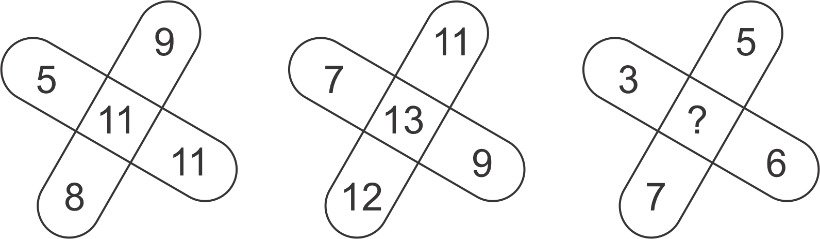Question of The Day19-11-2020

Insert the missing character depicted by ‘?’Correct Answer : d ) 7

Explanation :

Here, the logic in each figure is that one third of the sum of numbers at corners is equal to the number at center.

Considering first figure:

11=$$5+9+8+11 \over 3$$=$$33 \over 3$$

Considering second figure:

13=$$7+11+9+12 \over 3$$=$$39 \over 3$$=13

Considering third figure:

?=$$3+5+6+7 \over 3$$=$$21 \over 3$$=7

Hence, (d) is the correct answer.

Such type of question is asked in various government exams like SSC CGL, SSC MTS, SSC CPO, SSC CHSL, RRB JE, RRB NTPC, RRB GROUP D etc.

Read Daily Current Affairs, Banking Awareness, Hindi Current Affairs, Word of the Day, and attempt free mock tests at PendulumEdu and boost your preparation for the actual exam.0